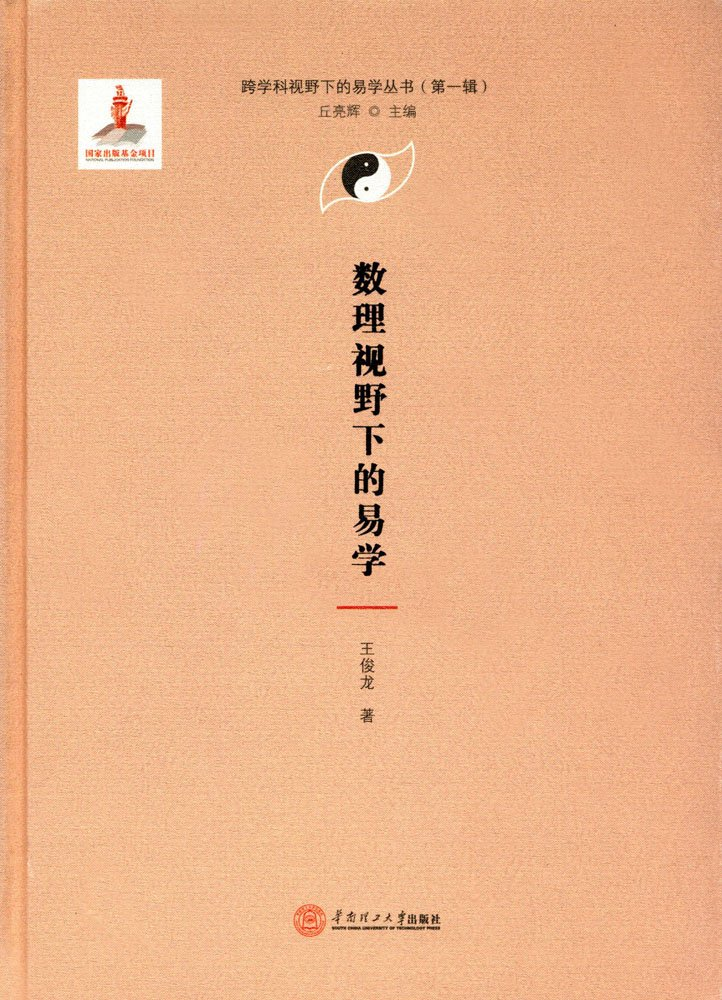DO NOT DELETE

# 古中醫學會圖書館藏書 Ancient Balance Medicine Association Library Collection

The first chapter explores the number of elephants
The first quarter is what is easy
One, easy to count
Second, easy to set is a group
Third, easy to set is a geometric model
Fourth, easy to set is binomial
V. Easy to collect and collect
Sixth, easy and matrix
Seven, easy-to-use and algebraic domains
The second part of the mathematical connotation of the number of students
First, the meaning of the number of major
Second, the number of major differences is the number of mutual multiplication
Third, the number of major gouache solution
4. The number of major differences is related to the nature and mathematics of divination.
Section III. Mathematics in Defamation Process, Order and Deduction
One, mathematics in the law of defamation
Second, the sequence of mathematics
Third, the interpretation of mathematics
The second chapter of the new law
The first section of the 爻 group (爻式) deduction method
I. The Eighth Palace Transmutation in Beijing
Second, the “Book of Changes” Tai Chi principle
Third, the special structure of the group
The second section of the matrix study
First, review of the classic matrix theory
Second, the construction of the matrix theory
Third, matrix representation of Shao Shao deduction method
Section III The Matrix Structure of the Quail Group
First, the nature of the matrix
Second, another way of expressing Fuxi Group
Third, the matrix representation of the parity deduction method
Fourth, the matrix structure of the group
Fifth, the ensemble group structure method
The third chapter is easy new certificate
Section 1 The Mathematical Essence of Qun Group
One, plus twice: a new solution to the theory of classical music
Second, the group deduction method: Introduction to modern theory
Section II Mathematical Proof of a Relevant Theorem
I. Gray code and its properties
Second, the mathematical nature of Yan Yiqun: Walsh function and its generation
Third, the recurrence theory and its proof
The Complete and Easy Interpretation of the Third Section Theorem and Its Proof
First, the definition and nature of the Walsh function
Second, the new application of Walsh function: block design
Third, Walsh function, block design and performance
Fourth, Walsh function and strengthen the relativity theory
The fourth chapter is the number of new knowledge
Section 1 Study on the Order of Groups
First, group order and number
Second, the order of the sequence and order group
Third, the order and replacement
Fourth, the invariance of cross ratio rules
The Second Section of Eighth Palace Number Theory and the Structure of Dai Group
The Structure of One and Eight Palaces and the Group
The two-octagon matrix and the Walsh function
Three, perfect sixteen yuan order group
The mathematical unity of the typical gossip sequence in the third quarter
First, the documentary records of the classic gossip sequence
Second, the table can be expressed as a mathematical function
The fifth chapter changes the new solution
Section 1 Intrinsic figure and periodic symmetry function
First, the nature of the periodic symmetry function
Second, the case analysis
The second section of the easy vector representation and its transformation
First, the easy mathematical connotation
Second, easy vector representation
Third, the transformation of the easy vector
IV. Diagonal matrix: transform operator
Section 6 6-order Operators: Sixty-four Shifts
One, no overturning, only change sign (main diagonal matrix)
Second, first inverted, post-transformation (subdiagonal matrix)
Third, pair transformation (I type): upside down, upside down transformation
Fourth, confrontation transformation (type II): squat down, upside down
Chapter 6: New Theory of Order
The first section of the current “Book of Changes” sequence structure and its interpretation
First, the structural analysis of this “Book of Changes” order
Second, today’s “Book of Changes” sequence deduction generation
III. Discussion: The status of SAR A and B in the current “Book of Changes” preface
A Preliminary Study on the Arrangement of Mathematical Rules in the Second Section of Today’s Book of Changes
First, sequence and sequence code
Second, the basic rules of order distribution
The Third Quarter: Revisiting the Mathematical Rules of the Book of Changes
First, discuss the distribution law of Yin and Wuyang
Second, discuss the distribution law of Sanyin Sanyang
Third, on the distribution law of the twelve rumor
IV. Ordinal Disposition Distribution
The fourth quarter of this book “Book of Changes” arranges the mathematics law
A mathematical representation of a special simple sequence and its general terms
Second, the order of the layout of the law and its general terms
Third, the principle of integration of sequence layout
Fourth, the further study of order and order
Section 5: The Coordinate Geometric Solution to the Distribution Rules of Preface and Miscellaneous in Zhouyi
First, hybrid and its code sequence
The construction of two, thirty-six grid checkerboard and Helu seventy-six square chart
Third, the art of straight lines: “Preface and Postscript”
Fourth, the creation of the plane: “mixed mantis” order three-dimensional geometric illustration
V. Discussion
references
indexNot Available

Similar Titles

"We cast a shadow on something wherever we stand, and it is no good moving from place to place to save things, because the shadow always follows. Choose a place where you won't do harm... yes, choose a place where you won't do very much harm, and stand in it for all you are worth, facing the sunshine." - E.M. Forster, A Room With A View

### (I Ching under the perspective of Mathematics and Science - The Series of I Ching Study under Transdisciplinary perspectives) 数理视野下的易学(精)/跨学科视野下的易学丛书

Author: 王俊龙
Pages: 192
Genre(s): Mathematics 數學 道象理數 算術
Publisher: South China University of Technology Press
Subject:
Country:
Publication Year: 2017
Editionfalse
Finished? No
Signed? No

HK\$ Donated by:

## 1 thought on “数理视野下的易学(精)/跨学科视野下的易学丛书 (I Ching under the perspective of Mathematics and Science – The Series of I Ching Study under Transdisciplinary perspectives) ISBN: 9787562352761”

1.Editor on said:

Recommended translation:
I Ching under the perspective of Mathematics and Science (The Series of I Ching Study under Transdisciplinary perspectives)